### # 什么是测试

1. 提高代码质量：测试就是找 BUG，找出 BUG，然后解决它。BUG 少了，代码质量自然就高了。
2. 可维护性：对现有代码进行修改、新增功能从而造成的成本越低，可维护性就越高。

### # 什么时候写测试

``````function add(a, b) {
return a + b
}

function sum(data = []) {
let result = 0
data.forEach(val => {
})

return result
}

console.log(sum([1,2,3,4,5,6,7,8,9,10])) // 55
``````

### # Jest

``````npm i -D jest
``````

``````"scripts": {
"test": "jest",
}
``````

## # 单元测试

1. 根据正确性写测试，即正确的输入应该有正常的结果。
2. 根据错误性写测试，即错误的输入应该是错误的结果。

### # 对一个函数做测试

``````// main.js
function abs(a) {
if (typeof a != 'number') {
throw new TypeError('参数必须为数值型')
}

if (a < 0) return -a
return a
}

// test.spec.js
test('abs', () => {
expect(abs(1)).toBe(1)
expect(abs(0)).toBe(0)
expect(abs(-1)).toBe(1)
expect(() => abs('abc')).toThrow(TypeError) // 类型错误
})
``````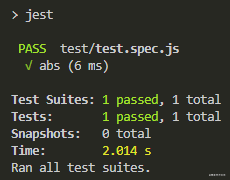### # 对一个类做测试

``````class Math {
abs() {

}

sqrt() {

}

pow() {

}
...
}
``````

``````test('Math.abs', () => {
// ...
})

test('Math.sqrt', () => {
// ...
})

test('Math.pow', () => {
// ...
})
``````

### # 对一个组件做测试

1. 点击 `<input type="file" />`，选择图片上传。
2. 触发 `input``change` 事件，获取 `file` 对象。
3. `FileReader` 将图片转换成 base64 码。

``````document.querySelector('input').onchange = function fileChangeHandler(e) {
const file = e.target.files
const fileResult = res.target.result
console.log(fileResult) // 输出 base64 码
}

}
``````

``````document.querySelector('input').onchange = function fileChangeHandler(e) {
const file = e.target.files
tobase64(file)
}

function tobase64(file) {
return new Promise((resolve, reject) => {
const fileResult = res.target.result
resolve(fileResult) // 输出 base64 码
}

})
}
``````

``````// 重写 File
window.File = function () {}

target: {
result: fileData,
},
})
}
}
``````

``````// 提前写好文件内容
const fileData = 'data:image/test'

// 提供一个假的 file 对象给 tobase64() 函数
function test() {
const file = new File()
const event = { target: { files: [file] } }
file.type = 'image/png'
file.name = 'test.png'
file.size = 1024

it('file content', (done) => {
tobase64(file).then(base64 => {
expect(base64).toEqual(fileData) // 'data:image/test'
done()
})
})
}

// 执行测试
test()
``````

### # 测试覆盖率

``````"scripts": {
"test": "jest --coverage",
}
``````

``````// main.js
function abs(a) {
if (typeof a != 'number') {
throw new TypeError('参数必须为数值型')
}

if (a < 0) return -a
return a
}

// test.spec.js
test('abs', () => {
expect(abs(1)).toBe(1)
expect(abs(0)).toBe(0)
expect(abs(-1)).toBe(1)
expect(() => abs('abc')).toThrow(TypeError) // 类型错误
})
``````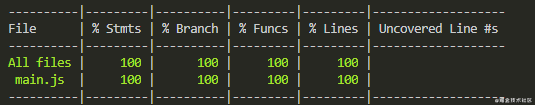``````// test.spec.js
test('abs', () => {
expect(abs(1)).toBe(1)
expect(abs(0)).toBe(0)
expect(abs(-1)).toBe(1)
// expect(() => abs('abc')).toThrow(TypeError)
})
``````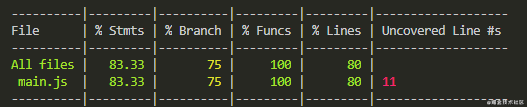``````// 就是这一个分支语句
if (typeof a != 'number') {
throw new TypeError('参数必须为数值型')
}
``````

#### # 覆盖率统计项

1. Stmts(statements)：语句覆盖率，程序中的每个语句是否都已执行。
2. Branch：分支覆盖率，是否执行了每个分支。
3. Funcs：函数覆盖率，是否执行了每个函数。
4. Lines：行覆盖率，是否执行了每一行代码。

``````if (typeof a != 'number') {
throw new TypeError('参数必须为数值型')
}

if (typeof a != 'number') throw new TypeError('参数必须为数值型')
``````

``````// expect(() => abs('abc')).toThrow(TypeError)
``````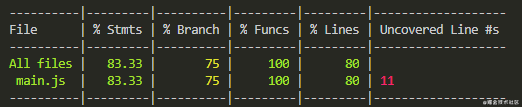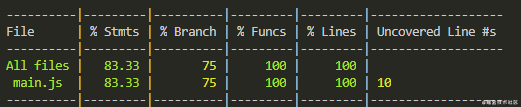### # TDD 测试驱动开发

TDD(Test-Driven Development) 就是根据需求提前把测试代码写好，然后根据测试代码实现功能。

TDD 的初衷是好的，但如果你的需求经常变（你懂的），那就不是一件好事了。很有可能你天天都在改测试代码，业务代码反而没怎么动。

## # E2E 测试

Cypress 在进行 E2E 测试时，会打开 Chrome 浏览器，然后根据测试代码对页面进行操作，就像一个正常的用户在操作页面一样。

### # 安装

``````npm i -D cypress
``````

``````"cypress": "cypress open"
``````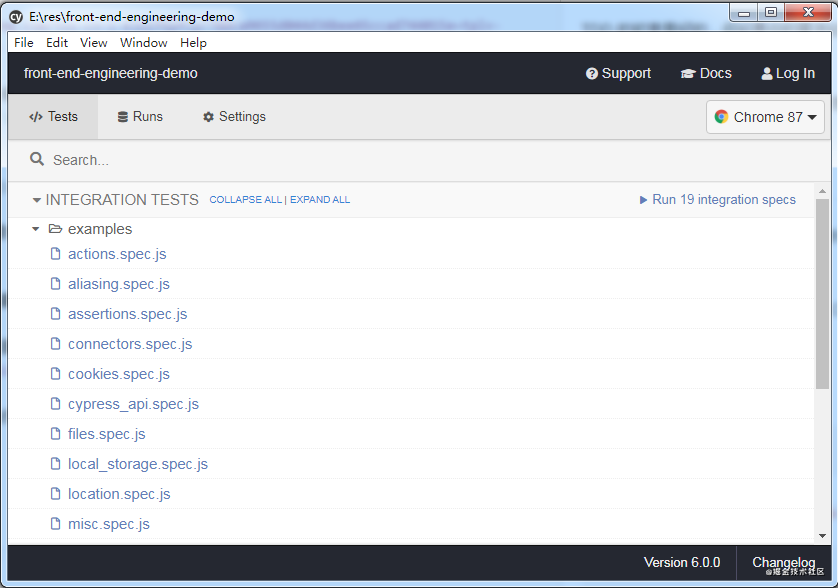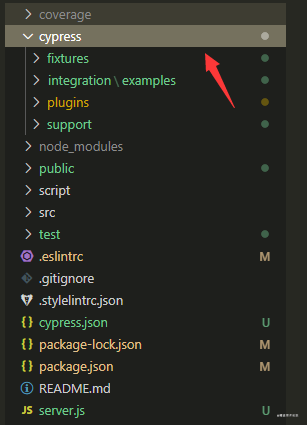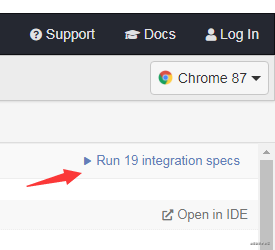### # 第一次测试

``````describe('The Home Page', () => {
cy.visit('http://localhost:8080')
})
})
``````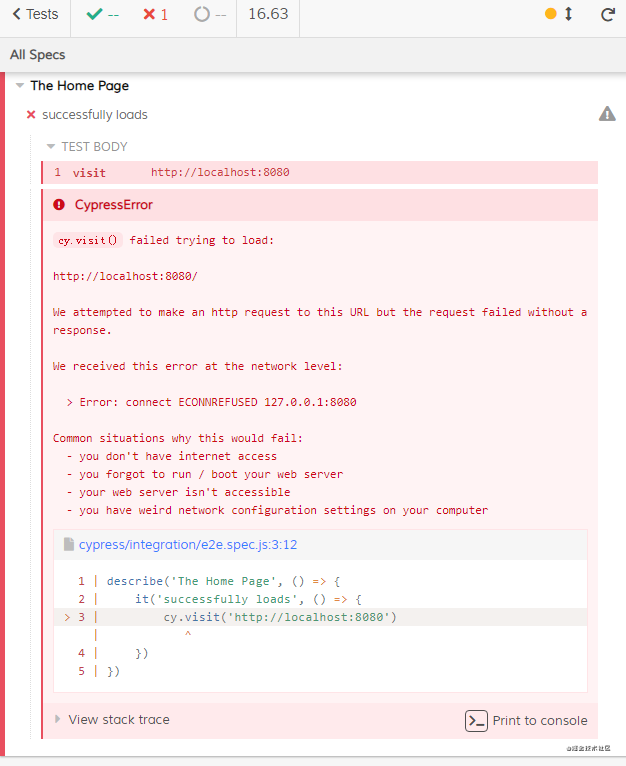``````// server.js
const express = require('express')
const app = express()
const port = 8080

app.get('/', (req, res) => {
res.send('Hello World!')
})

app.listen(port, () => {
console.log(`Example app listening at http://localhost:\${port}`)
})
``````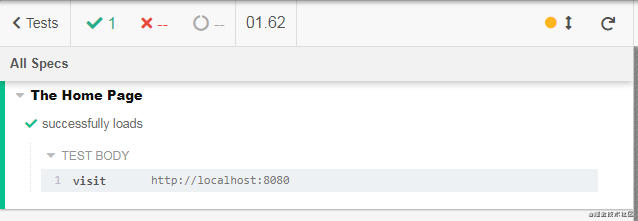PS： 如果你使用了 ESlint 来校验代码，则需要下载 `eslint-plugin-cypress` 插件，否则 Cypress 的全局命令会报错。下载插件后，打开 `.eslintrc` 文件，在 `plugins` 选项中加上 `cypress`

``````"plugins": [
"cypress"
]
``````

### # 模仿用户登录

1. 用户打开登录页 `/login.html`
2. 输入账号密码（都是 `admin`
3. 登录成功后，跳转到 `/index.html`

``````// server.js
const bodyParser = require('body-parser')
const express = require('express')
const app = express()
const port = 8080
app.use(express.static('public'))
app.use(bodyParser.urlencoded({ extended: false }))
app.use(bodyParser.json())

const { account, password } = req.body
res.send({
msg: '登录成功',
code: 0,
})
} else {
res.send({
msg: '登录失败，请输入正确的账号密码',
code: 1,
})
}
})

app.listen(port, () => {
console.log(`Example app listening at http://localhost:\${port}`)
})
``````

``````<!-- login.html  -->
<!DOCTYPE html>
<html lang="en">
<meta charset="UTF-8">
<meta name="viewport" content="width=device-width, initial-scale=1.0">
<style>
div {
text-align: center;
}

button {
display: inline-block;
line-height: 1;
white-space: nowrap;
cursor: pointer;
text-align: center;
box-sizing: border-box;
outline: none;
margin: 0;
transition: 0.1s;
font-weight: 500;
font-size: 14px;
color: #fff;
background-color: #409eff;
border-color: #409eff;
border: 0;
}

button:active {
background: #3a8ee6;
border-color: #3a8ee6;
color: #fff;
}

input {
display: block;
margin: auto;
margin-bottom: 10px;
-webkit-appearance: none;
background-color: #fff;
background-image: none;
border: 1px solid #dcdfe6;
box-sizing: border-box;
color: #606266;
font-size: inherit;
height: 40px;
line-height: 40px;
outline: none;
transition: border-color 0.2s cubic-bezier(0.645, 0.045, 0.355, 1);
}
</style>
<body>
<div>
<input type="text" placeholder="请输入账号" class="account">
<button>登录</button>
</div>
<script src="https://cdn.bootcdn.net/ajax/libs/axios/0.21.0/axios.min.js"></script>
<script>
document.querySelector('button').onclick = () => {
account: document.querySelector('.account').value,
})
.then(res => {
if (res.data.code == 0) {
location.href = '/index.html'
} else {
}
})
}
</script>
</body>
</html>
``````
``````<!-- index.html  -->
<!DOCTYPE html>
<html lang="en">
<meta charset="UTF-8">
<meta name="viewport" content="width=device-width, initial-scale=1.0">
<title>index</title>
<body>
Hello World!
</body>
</html>
``````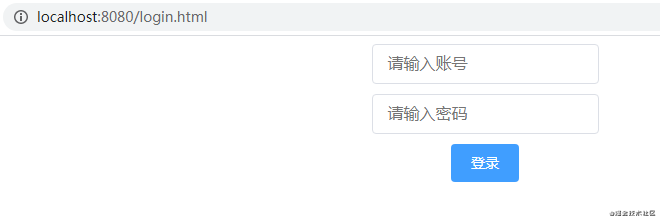index.html 静态页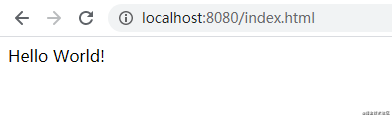``````describe('The Home Page', () => {
// 输入账号密码

cy.get('button').click()
// 重定向到 /index
cy.url().should('include', 'http://localhost:8080/index.html')

// 断言 index.html 页面是包含 Hello World! 文本
cy.get('body').should('contain', 'Hello World!')
})
})
``````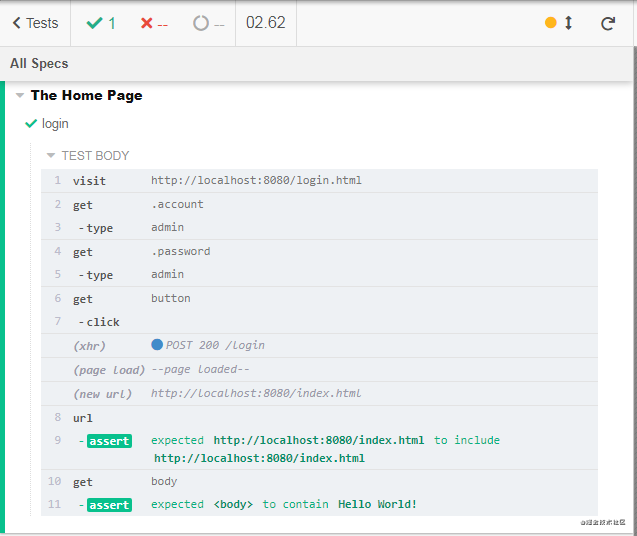``````"scripts": {
"test": "jest --coverage test/",
"lint": "eslint --ext .js test/ src/",
"start": "node server.js",
"cypress": "cypress open"
}
``````